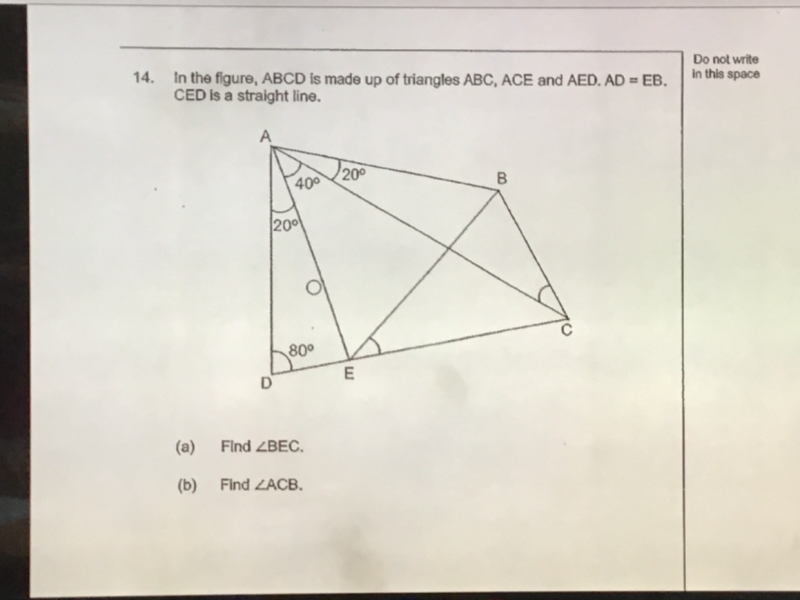# QuestionPls help to solve (b)

(a)  Angle AEB = 180-80-20 = 80 => AE = AD = EB

Angle BAC = 60  => Angle AEB = 60 (equilateral triangle AE = EB = AB)

Hence Angle BEC = 180 – 80-60  = 40  (Angles on a straight line)

(b) Angle AEC = 60 + 40 = 100  => Angle ACE = 40  => AE = EC = EB

Angle ECB = (180-40)/ 2  = 70 (angles in isoc triangle, EB=EC)

Therefore, Angle ACB = Angle ECB – Angle ACE = 70-40 = 300